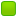###Author Topic: Elementary Number Theory Exercises  (Read 5808 times)

0 Members and 1 Guest are viewing this topic.

####Ender

• Moderator
• Hero Member
••• Posts: 2398##### Elementary Number Theory Exercises
« on: February 24, 2008, 08:05:05 pm »
Here's some exercises I made up while on my way and eating dinner at a restaurant =) No calculators allowed.

1. Find the remainder when 3^1337 is divided by 7.

2. Find the remainder when 13^37 is divided by 49.

I really like the second oneI think it's cooler.

Solutions posted.
« Last Edit: February 28, 2008, 08:18:37 am by Ender »

####Ender

• Moderator
• Hero Member
••• Posts: 2398##### Re: Elementary Number Theory Exercises
« Reply #1 on: February 24, 2008, 08:14:45 pm »
I forgot to say that you should, of course, post a solution with your answer.

EDIT: I will post the solution in a week on Thursday (a week is too long, and I would post it earlier but some people may not see the thread before then).
« Last Edit: February 24, 2008, 08:48:37 pm by Ender »

####rabbit

• x86
• Hero Member
••• Posts: 8105
• I speak for the entire clan (except Joe)##### Re: Elementary Number Theory Exercises
« Reply #2 on: February 25, 2008, 08:18:13 am »
The first is 1.

I don't feel like remembering how to do 13^37 :\

####Ender

• Moderator
• Hero Member
••• Posts: 2398##### Re: Elementary Number Theory Exercises
« Reply #3 on: February 25, 2008, 08:37:01 am »

I forgot to say that you should, of course, post a solution with your answer.

####rabbit

• x86
• Hero Member
••• Posts: 8105
• I speak for the entire clan (except Joe)##### Re: Elementary Number Theory Exercises
« Reply #4 on: February 25, 2008, 07:03:06 pm »

I forgot to say that you should, of course, post a solution with your answer.

####Sidoh

• Moderator
• Hero Member
••• Posts: 17668
• MHNATY ~~~~~##### Re: Elementary Number Theory Exercises
« Reply #5 on: February 25, 2008, 07:06:28 pm »

Mathematica says you're wrong too.Ender, I'll think about it a bit.

####Ender

• Moderator
• Hero Member
••• Posts: 2398##### Re: Elementary Number Theory Exercises
« Reply #6 on: February 28, 2008, 08:13:30 am »
Solution to 1:

Solution: We can use modular arithmetic and express our remainder as $$r = 3^{1337} \pmod{7}$$. Since 3 is coprime to 7, we can use Fermat's Little Theorem, and we find that $$3^6 \equiv 1 \pmod{7}$$. We see that $$6 | 1332$$ since 1332 is even and 1+3+3+2=9. It follows that that for some integer k, we have $$r = 3^{1337} = 3^{6k + 5} = 3^{6k} * 3^{5} \equiv 1 * 3^{5} \pmod{7}$$, greatly simplifying the problem.

For the last step,

$$r = 3^{5} = \frac{3^{6}}{3} \equiv \frac{1}{3} \pmod{7}$$. Thus $$3r \equiv 1 \pmod{7}$$. By observation we see that r=5 satisfies this linear congruence, so the remainder is 5.

This looks long because I wrote out all the steps, but it can be done in the head pretty fast. Another way to do this is to make a table of remainders for small powers until it repeats. Since this table will be periodic, you can predict the remainder for larger powers. This alternate way doesn't require much knowledge of number theory.

####Ender

• Moderator
• Hero Member
••• Posts: 2398##### Re: Elementary Number Theory Exercises
« Reply #7 on: February 28, 2008, 08:37:11 am »
Solution to 2:

We have $$r = 13^{37} \pmod{49}$$. Note that 13 is coprime to 49 and that $$\phi(49) = 7^{2} - 7 = 42$$. By Euler's Theorem, we can simplify this to $$r \equiv 13^{-5} \pmod{49}$$ and so $$13^{5}r \equiv 1 \pmod{49}$$. We can calculate $$13^{2} \equiv 22 \pmod{49}$$ to get $$13^{4} \equiv -6 \pmod{49}$$ and finally $$13^{5} \equiv 20 \pmod{49}$$. Thus we have $$13^{5} r \equiv 20 r \equiv 1 \pmod{49}$$. This seems like a hard linear congruence to solve, but it's much easier if you notice that 9*49 must be one more than a multiple of 20, since 40 is a multiple of 20 and 9*9 = 80 + 1. We have 9*49 = 360 + 81 = 441, so $$20*22 \equiv -1 \pmod{49}$$ shows us that $$20*(-22) \equiv 1 \pmod{49}$$. Making that positive, we see that r = 27.

I actually meant to make this problem easier, but when I did it out I saw that there were some computational obstacles (which still can be done by hand fairly easily, as is what I did). I may have made an arithmetic mistake though, so it would be cool if someone checked this on Mathematica. So for a followup problem:

What is the remainder when you divide 13^43 by 49?

This problem has a one-line solution. Hint: Euler's Theorem.
« Last Edit: February 28, 2008, 09:33:10 am by Ender »

####Ender

• Moderator
• Hero Member
••• Posts: 2398##### Re: Elementary Number Theory Exercises
« Reply #8 on: March 01, 2008, 11:46:22 am »
What is the remainder when you divide 13^43 by 49?

Solution. $$\phi(49) = 7^{2} - 7 = 42$$. Since 13 is coprime to 49, we can employ Euler's Theorem, and we find that $$13^{42} \equiv 1 \pmod{49}$$. It follows that $$13^{43} \equiv 13 \pmod{49}$$, so 13 is the remainder.

This is what is considered a "one-liner" in math. If you want, it can be written in one-line with less clarification